In :
import json
import matplotlib
s = json.load( open("styles/qutip_matplotlibrc.json") )  #edit path to json file
matplotlib.rcParams.update(s)

In :
%pylab inline

Populating the interactive namespace from numpy and matplotlib

In :
from IPython.display import Image, HTML

In :
HTML('''<script>
code_show=true;
function code_toggle() {
if (code_show){
$('div.input').hide(); } else {$('div.input').show();
}
code_show = !code_show
}
</script>
The raw code for this IPython notebook is by default hidden for easier reading.
To toggle on/off the raw code, click <a href="javascript:code_toggle()">here</a>.''')

Out:
The raw code for this IPython notebook is by default hidden for easier reading. To toggle on/off the raw code, click here.

# Plotting in Python Using Matplotlib¶

P.D. Nation and J.R. Johansson

## Introduction¶

Being able to plot high-quality, informative figures is one of the necessary tools for working in the sciences today. If your figures don't look good, then you don't look good. Good visuals not only help to convey scientific information, but also help to draw attention to your work. Often times good quality figures and plots play an important role in determining the overall scientific impact of your work. Therefore we will spend some time learning how to create high-quality, publication ready plots in Python using a Python module called Matplotlib.

In :
Image(filename='images/mpl.png',width=700,embed=True)

Out:## Basic 2D Plotting¶

Before generating plots in Python we must first load the main Matplotlib module.

In :
from pylab import *


Let us also import numpy so we can use arrays and mathematical functions

In :
from numpy import *


### Drawing Lines¶

Plotting a simple function, such as a sine function, is easy to do. All we need are two arrays, one for the x values, and one for the f(x) values

In :
x=linspace(-pi,pi)
y=sin(x)
plot(x,y)
show()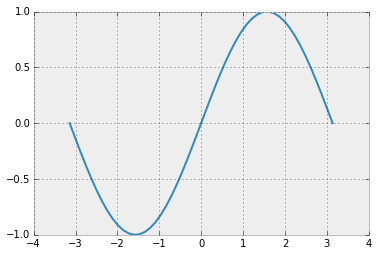Here, the plot command generates the figure, but it is not displayed until you run show(). If we want, we can also also add some labels to the axes and a title to the plot. While we are at it, lets change the color of the line to red, and make it a dashed line.

In :
x=linspace(-pi,pi)
y=sin(x)
plot(x,y,'r--') #make line red 'r' and dashed '--'
xlabel('x')
ylabel('y')
title('sin(x)')
show()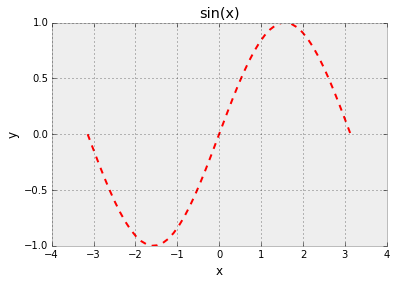Here the 'r' stands for red, but we could have used any of the built in colors:

• 'b': blue
• 'g': green
• 'r': red
• 'c': cyan
• 'm': magenta
• 'y': yellow
• 'k': black
• 'w': white

We can also specify the color of a line using the color keyword argument

In :
x=linspace(-pi,pi)
y=sin(x)
plot(x,y,'--',color='0.75') # Here a string from 0->1 specifies a gray value.
show()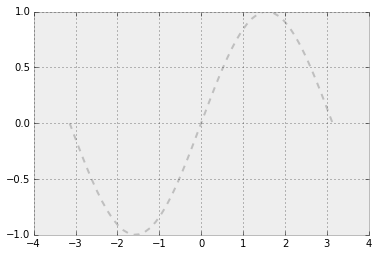In :
x=linspace(-pi,pi)
y=sin(x)
plot(x,y,'-',color='#FD8808') # We can also use hex colors if we want.
show()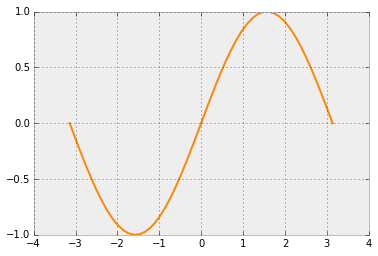The style of the line can be changed from solid: '' or '-', to dashed: '--', dotted '.', dash-dotted: '-.', dots+solid: '.-', or little dots: ':'. One can also use the 'linstyle' or 'ls' keyword argument to change this style. We can disply all of these variations using the subplot function that displays multiple plots in a grid specified by the number of rows, columns, and the number of the current plot. We only need one show() command for viewing all of the plots. To make the plot look good, we can also control the width and height of the figure by calling the figure function using the keyword argument 'figsize' that specifies the width and height of the figure in inches.

In :
x=linspace(-pi,pi)
y=sin(x)
figure(figsize=(12,3)) #This controls the size of the figure
subplot(2,3,1) #This is the first plot in a 2x3 grid of plots
plot(x,y)
subplot(2,3,2)#this is the second plot
plot(x,y,linestyle='--') # Demo using 'linestyle' keyword arguement
subplot(2,3,3)
plot(x,y,'.')
subplot(2,3,4)
plot(x,y,'-.')
subplot(2,3,5)
plot(x,y,'.-')
subplot(2,3,6)
plot(x,y,ls=':') # Demo using 'ls' keyword arguement.
show()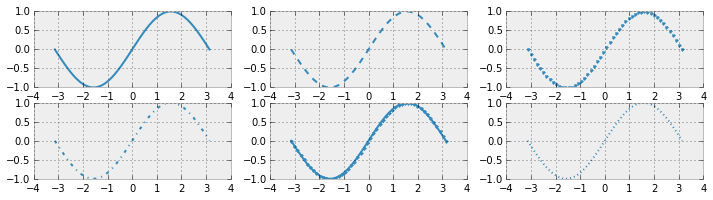If we want to change the width of a line then we can use the 'linewidth' or 'lw' keyword arguements with a float number specifying the linewidth

In :
x=linspace(0,10)
y=sqrt(x)
figure(figsize=(12,3))
subplot(1,3,1)
plot(x,y)
subplot(1,3,2)
plot(x,y,linewidth=2)
subplot(1,3,3)
plot(x,y,lw=7.75)
show()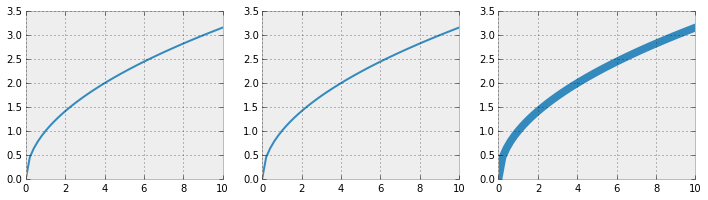If we want to plot multiple lines on a single plot, we can call the plot command several times, or we can use just a single plot command by entering the data for multiple lines simultaneously.

In :
x=linspace(0,10)
s=sin(x)
c=cos(x)
sx=x*sin(x)
figure(figsize=(12,3))
subplot(1,2,1)
plot(x,s,'b')    #call three different plot functions
plot(x,c,'r')
plot(x,sx,'g')

subplot(1,2,2)
plot(x,s,'b',x,c,'r',x,sx,'g') #combine multiple lines in one call to plot
show()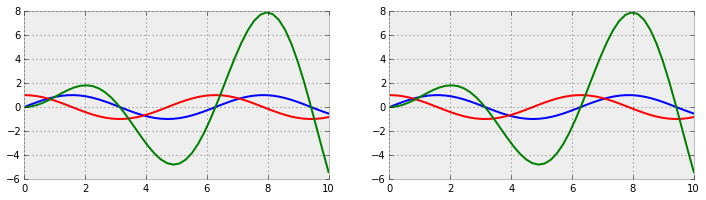### Setting Axes Limits¶

Suppose we want to limit the range of either the x-axis or y-axis, or both. In Matplotlib we can use the xlim and ylim functions to accomplish this.

In :
x=linspace(-pi,pi,100)
figure(figsize=(12,3))
subplot(1,3,1)
plot(x,sin(x),lw=2)
subplot(1,3,2)
plot(x,sin(x),lw=2,color='#740007')
xlim([-pi,pi])                       #change bounds on x-axis to [-pi,pi]
subplot(1,3,3)
plot(x,sin(x),'^',ms=8,color='0.8')
xlim([-1,1])                         #change bounds on x-axis to [-1,1]
ylim([-0.75,0.75])                   #change bounds on y-axis to [-0.75,0.75]
show()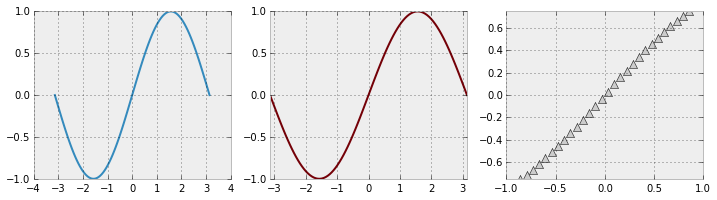### Saving Figures¶

Now that we know how to make good looking plots, it would certainly be nice if we knew how to save these figures for use in a paper/report, or perhaps for posting on a webpage. Fortunately, this is very easy to do. If we want to save our previous figure then we need call the savefig function.

In :
x=linspace(-pi,pi,100)
figure(figsize=(12,3))
subplot(1,3,1)
plot(x,sin(x),lw=2)
subplot(1,3,2)
plot(x,sin(x),lw=2,color='#740007')
xlim([-pi,pi])
subplot(1,3,3)
plot(x,sin(x),'^',ms=8,color='0.8')
xlim([-1,1])
ylim([-0.75,0.75])
savefig('axes_example.png') #Save the figure in PNG format in same directory as script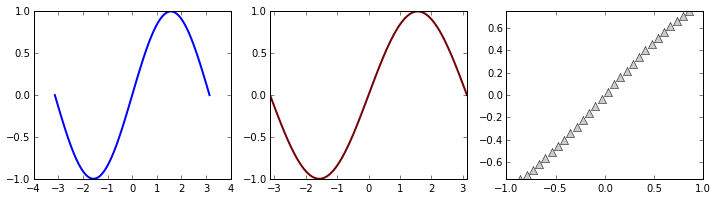savefig saves the figure with the name and extension that is given in the string. The name can be whatever you like, but the extension. .png in this case, must be a format that Matplotlib recognizes. In this class we will only use the Portable Network Graphics .png and PDF .pdf formats.

### Using Shapes for Data Points¶

In addition to lines and dots, Matplotlib allows you to use many different shapes to represent the points on a graph. In Matplotlib, these shapes are called markers and just like lines, their color and size can be controlled. There are many basic types of markers, so here we will demonstrate just a few important ones: '*': star, 'o': circle, 's': square, and '+': plus by evaluating the Airy functions.

In :
from scipy.special import airy #Airy functions are in the SciPy special module
x = linspace(-1,1)
Ai,Aip,Bi,Bip = airy(x)
plot(x,Ai,'b*',x,Aip,'ro',x,Bi,'gs',x,Bip,'k+')
show()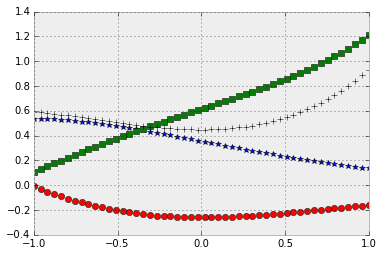We can also chage the size of the shapes using the 'markersize' or 'ms' keyword arguments, and the color using 'markercolor' or 'mc'.

## Other Kinds of 2D Plots¶

So far we have made use of only the plot function for generating 2D figures. However, there are several other functions for generating different kinds of 2D plots. A collection of the many different types can be found at the matplotlib gallery, and we will highlight only the more useful functions.

In :
x = np.linspace(-1, 1., 100)
figure(figsize=(6,6))
subplot(2,2,1)
y=x+0.25*randn(len(x))
scatter(x,y,color='r') #plot collection of (x,y) points
title('A scatter Plot')
subplot(2,2,2)
n = array([0,1,2,3,4,5])
bar(n, n**2, align="center", width=1) #aligns the bars over the x-numbers, and width=dx
title('A bar Plot')
subplot(2,2,3)
fill_between(x, x**2, x**3, color="green") #fill between x**2 & x**3 with green
title('A fill_between Plot')
subplot(2,2,4)
title('A hist Plot')
r=random.randn(50) # generating some random numbers
hist(r,color='y') #create a histogram of the random number values
show()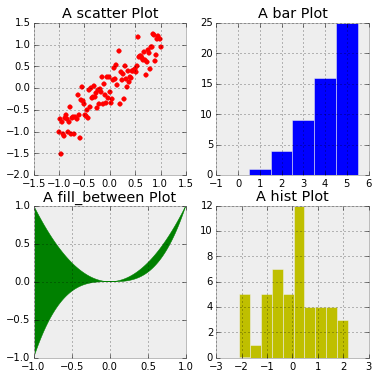The color and size of the elements can all be controlled in the same way as the usual plot function.

# End of Tutorial

from IPython.core.display import HTML